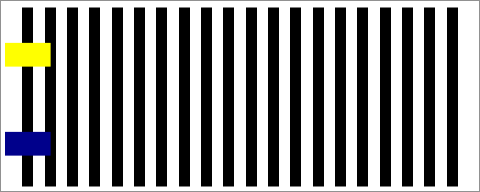## Fun with SAS ODS Graphics: Stepping Feet IllusionStepping Feet Illusion

With the stepping feet illusion racking up likes on Twitter this week, thought I'd take a crack at recreating it using SAS ODS Graphics!``````* Fun w/SAS ODS Graphics: Stepping feet illusion (see en.wikipedia.org/wiki/Stepping_feet_illusion);

data barsNmarkers;                      * Generate points for black lines and yellow & blue markers;
do frame=1 to 20 by .1;                 * Move markers between x=2 and x=18 in increments of .1;
y=1;
do x=1 to 20;                         * Draw 20 lines 1 unit high;
output;
end;
x=frame; y=.;                         * Points for yellow and dark blue markers;
yy=.75; yb=.25; output; yy=.; yb=.;
end;

ods _all_ close;                        * Animated GIF setup;
options papersize=('5 in', '2 in') printerpath=gif animation=start
nodate nonumber animloop=YES NOANIMOVERLAY animduration=.075;
ods printer file='/folders/myfolders/SteppingFeet.gif';
ods graphics / width=5in height=2in imagefmt=GIF;

options nobyline;                       * Stepping feet illusion for first 13 bars (black bars create contrast);
proc sgplot data=barsNmarkers(where=(frame<14)) noautolegend noborder nowall;
by frame;
symbolchar name=uniRect char='25AC'x;   * Unicode for rectangle;
dropline x=x y=y / lineattrs=(color=black thickness=11pt);
scatter x=x y=yy / markeroutlineattrs=(color=yellow) markerattrs=(color=yellow size=144pt symbol=uniRect);
scatter x=x y=yb / markeroutlineattrs=(color=darkblue) markerattrs=(color=darkblue size=144pt symbol=uniRect);
xaxis display=none offsetmin=0 offsetmax=0 min=0.5 max=21 type=linear;
yaxis display=none offsetmin=0 offsetmax=0 min=0 max=1;

* No Stepping feet illusion for last 7 bars (white bars create no contrast);
proc sgplot data=barsNmarkers(where=(frame>=14)) noautolegend noborder nowall;
by frame;
symbolchar name=uniRect char='25AC'x;   * Unicode for rectangle;
dropline x=x y=y / lineattrs=(color=white thickness=11pt);
scatter x=x y=yy / markeroutlineattrs=(color=yellow) markerattrs=(color=yellow size=144pt symbol=uniRect);
scatter x=x y=yb / markeroutlineattrs=(color=darkblue) markerattrs=(color=darkblue size=144pt symbol=uniRect);
xaxis display=none offsetmin=0 offsetmax=0 min=0.5 max=21 type=linear;
yaxis display=none offsetmin=0 offsetmax=0 min=0 max=1;
run;

options printerpath=gif animation=stop; * Animated GIF wrapup;
ods printer close;``````
2 REPLIES 2

## Re: Fun with SAS ODS Graphics: Stepping Feet Illusion

Brilliant @tc

//Contact me to learn how Metacoda software can help keep your SAS platform secure - https://www.metacoda.comKsharp
Super User

## Re: Fun with SAS ODS Graphics: Stepping Feet Illusion

I like using symbolchar statement to draw a rectangle ,

and dropline statement to repalace block statement

Discussion stats
• 2 replies
• 1551 views
• 13 likes
• 3 in conversation